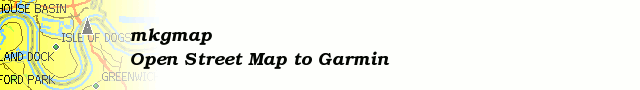# [mkgmap-dev] DEM format Questions

From Gerd Petermann gpetermann_muenchen at hotmail.com on Fri Nov 10 11:10:04 GMT 2017

```Hi Frank,

my problem is that I don't understand the pdf. When I try to read it my brain gets woozy, so I started to trace what TileEncoder.cs is doing using an input file for an area in Niedersachsen and
in parallel I try to code a bitstream reader in DemDisplay.
I still have no clue how the reader knows in what way the numbers are coded.
I think I undetstand the section with header "Datenberechnung", but the following "Standardwerte" is still a miracle...

Gerd

________________________________________
Von: mkgmap-dev <mkgmap-dev-bounces at lists.mkgmap.org.uk> im Auftrag von Frank Stinner <frank.stinner at leipzig.de>
Gesendet: Freitag, 10. November 2017 11:48:53
An: mkgmap-dev at lists.mkgmap.org.uk
Betreff: Re: [mkgmap-dev] DEM format Questions

Hi Gerd,

which 0-Bit you mean? Let us take this example:

4,4,4,5,7,7,7,7,7,7,7,7,7,7,7,7,7,7,7,7,7,7,7,7,7,7,7,7,7,7,7,7,7,7,7,7,7,7,7,7,7,7,7,7,7,7,7,7,7,7,7,7,7,7,7,7,7,7,7,7,7,7,7,7
8,8,5,5,5,5,5,8

The detailview from my prog "Input2" (https://github.com/FSofTlpz/Garmin-DEM-Build/blob/master/Input2/bin/Input2.exe) show us:

MaxHeigth=158, Codingtype=0, TileSize=64x64, BaseHeigthUnit=1, HeigthUnit=0, ActualMode=notdefined, ActualHeigth=0
Line 0
Idx=0, Plateau Length=0 TableIdx=0 Bits=0 [.] <>
Idx=0, PlateauFollower ActualHeigth=4, Value=4 PlateauFollower0 [...11] Hybrid1 ddiff=0
Idx=1, ActualHeigth=4, Value=0 Standard [1.] Hybrid1
...
Idx=62, ActualHeigth=7, Value=0 Standard  Length0
Idx=63, ActualHeigth=7, Value=0 Standard  Length0
Line 1
Idx=0, Plateau Length=0 TableIdx=0 Bits=0 [.] <>
Idx=0, PlateauFollower ActualHeigth=8, Value=4 PlateauFollower0 [.111] Hybrid2 ddiff=0
Idx=1, ActualHeigth=8, Value=0 Standard  Length0
Idx=2, ActualHeigth=5, Value=-3 Standard [......1] Length0
Idx=3, Plateau Length=4 TableIdx=3 Bits=1 [1111..] <111a>
Idx=7, PlateauFollower ActualHeigth=8, Value=-1 PlateauFollower1 [.1.] Hybrid1 ddiff=2

Between the brackets [ and ] are the bits (. means 0).

There is no special case. If a plateau have to begin, we have as first the plateau-length (see "Codierung der Plateaulänge"). There is ever a 0-Bit between the 2 parts of length-coding, also when plateau-length=0 (often on start of a line).
As next we have the height-coding for the follower.

Frank
```